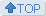全部 | 精品 | 发贴 刷新
1. <JoKwon*Gain>=消息=亚当夫妇 想...

2. <Jokwon*Gain>=图文=我们结婚了，...

3. <jokwon*gain>=消息=</jokw...

4. <JoKwon*Gain>=消息=谁和我唠唠亚...

5. <JoKwon*Gain>=消息=亚当夫妇十周...

6. <JoKwon*Gain>=图文=09年至现在...

7. <JoKwon*Gain>=消息=好几年没有

8. <JoKwon*Gain>=格式=权父母家有两...[]

9. <JoKwon*Gain>=消息=2020了

10. <Jokwon*Gain>=图文=挽救细节

11. <Jokwon*Gain>=图文=铁一般的证据...

12. <JoKwon*Gain>=消息=这两天在家

13. <JoKwon*Gain>=消息=一直在逛亚

14. <JoKwon*Gain>=消息＝為什麼權兒

15. <JoKwon*Gain>=消息=赵权Mwav...

16. <Jokwon*Gain>=图文=关于亚当的歪...

17. <Jokwon*Gain>=文文=我们再婚了[]

18. <JoKwon*Gain>=消息=赵权201

19. <JoKwon*Gain>=文文=我会抓住你

20. <JoKwon*Gain>=娱乐=亚当故事大接...2020-6-01 09:41